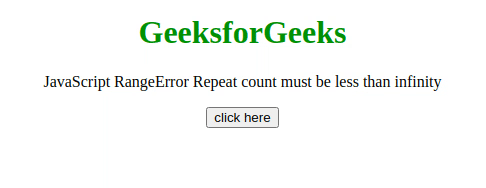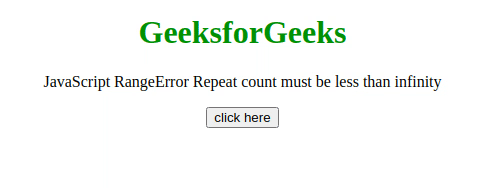# JavaScript RangeError – Repeat count must be less than infinity

This JavaScript exception repeat count must be less than infinity occurs if the passed argument of String.prototype.repeat() method is infinity.

Message:

```RangeError: argument out of range (Edge)
RangeError: repeat count must be less than infinity and not
overflow maximum string size (Firefox)
RangeError: Invalid count value (Chrome)```

Error Type:

`RangeError`

Cause of the error: The count parameter of String.prototype.repeat() method passed is either less than 0 or greater than infinity.

Example 1: In this example, the parameter passed is 4, So the error has not occurred.

## HTML

 ` ` `<``html``> ` `    ``<``body` `style``=``"text-align: center;"``> ` `        ``<``h1` `style``=``"color: green;"``> ` `            ``GeeksforGeeks ` `        `` ` ` `  `        ``<``p``> ` `            ``JavaScript RangeError ` `            ``Repeat count must be less than infinity ` `        `` ` ` `  `        ``<``button` `onclick``=``"Geeks();"``> ` `            ``click here ` `        `` ` ` `  `        ``<``p` `id``=``"GFG_DOWN"``> ` ` `  `        ``<``script``> ` `            ``var el_down = document.getElementById("GFG_DOWN"); ` ` `  `            ``function Geeks() { ` `                ``try { ` `                    ``"GFG".repeat(4); ` `                    ``el_down.innerHTML = "'Repeat count " + ` `                      ``"must be less than infinity' " + ` `                      ``"error has not occurred"; ` `                ``} catch (e) { ` `                    ``el_down.innerHTML = "'Repeat count " + ` `                      ``"must be less than infinity' " +  ` `                      ``"error has occurred"; ` `                ``} ` `            ``} ` `        `` ` `    `` ` ` `

Output:Example 2: In this example, the parameter passed is 232, So the error has occurred,

## HTML

 ` ` `<``html``> ` `    ``<``body` `style``=``"text-align: center;"``> ` `        ``<``h1` `style``=``"color: green;"``> ` `            ``GeeksforGeeks ` `        `` ` ` `  `        ``<``p``> ` `            ``JavaScript RangeError  ` `            ``Repeat count must be less than infinity ` `        `` ` ` `  `        ``<``button` `onclick``=``"Geeks();"``> ` `            ``click here ` `        `` ` ` `  `        ``<``p` `id``=``"GFG_DOWN"``> ` ` `  `        ``<``script``> ` `            ``var el_down = document.getElementById("GFG_DOWN"); ` `            ``function Geeks() { ` `                ``try { ` `                    ``"GFG".repeat(2 ** 32); ` `                    ``el_down.innerHTML = "'Repeat count " + ` `                      ``"must be less than infinity' " +  ` `                      ``"error has not occurred"; ` `                ``} catch (e) { ` `                    ``el_down.innerHTML = "'Repeat count " + ` `                      ``"must be less than infinity' " +  ` `                      ``"error has occurred"; ` `                ``} ` `            ``} ` `        `` ` `    `` ` ` `

Output:My Personal Notes arrow_drop_upCheck out this Author's contributed articles.

If you like GeeksforGeeks and would like to contribute, you can also write an article using contribute.geeksforgeeks.org or mail your article to contribute@geeksforgeeks.org. See your article appearing on the GeeksforGeeks main page and help other Geeks.

Please Improve this article if you find anything incorrect by clicking on the "Improve Article" button below.

Article Tags :

Be the First to upvote.

Please write to us at contribute@geeksforgeeks.org to report any issue with the above content.